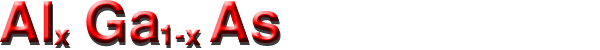## Mobility and Hall Effect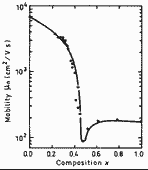Electron Hall mobility versus alloy composition x. T=300 K. Electron concentration no=(5÷10)·1015 cm-3. (Saxena (1981b).

#### For weakly doped AlxGa1-xAs at 300 K electron Hall mobility.

0<x<0.45   µH=-8000 - 22000x+ 10000x2
0.45<x<1   µH=-255+1160x-720x2
(Shur (1990)).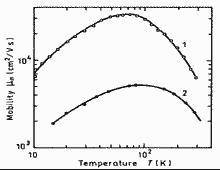Electron Hall mobility versus temperature. Curve 1 x=0; n=0.5·1016 cm-3 (Stillman et al. (1970)). Curve 2 x=0.32; n=(0.5÷1)·1016 cm-3 (Saxena (1981b)).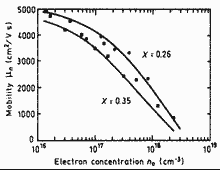Electron Hall mobility versus electron concentration for two values of x. T=77 K. (Liu (1990)).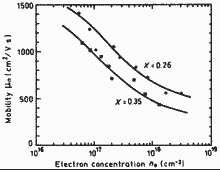Electron Hall mobility versus electron concentration for two values of x. T=300 K. (Liu (1990)).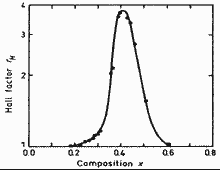Hall factor versus alloy composition x for n-type AlxGa1-xAs T=300 K Electron concentration no=(5÷10)·1015 cm-3. (Saxena (1981a).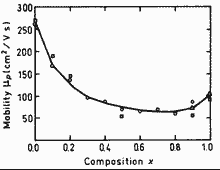Hole Hall mobility versus alloy composition x. 296 K. Acceptor density Na≈2.5·1017 cm-3. (Look et al. (1992)).

#### For weakly doped AlxGa1-xAs at 300 K hole Hall mobility.

µH=-370-970x+740x2
(Shur (1990)).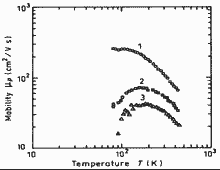Hole Hall mobility versus temperature. Curve 1 - x=0; po=7·1017 cm-3 Curve 2 - x=0.41; po=4.65·1017 cm-3 Curve 3 - x=0.75; po=2.4·1017 cm-3 (Yang et al. (1981)).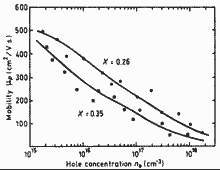Hole Hall mobility versus hole concentration for two values of x. T=77 K. (Liu (1990)).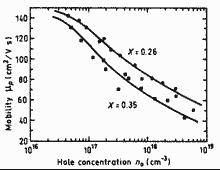Hole Hall mobility versus hole concentration for two values of x. T=300 K. (Liu (1990)).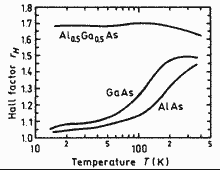Hall factor versus temperature x for p-type GaAs, AlAs and Al0.5Ga0.5As. Curves are calculated for acceptor concentration Na≈6.5·1013 cm-3. (Look et al. (1992)).